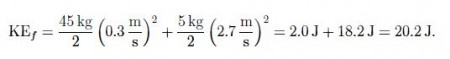# Momentum: Mathematical Solution

Example : A person [mass mp = 43 kg] is standing on a skateboard [mass mb = 2 kg ] at rest, while holding a dumbbell [mass M = 5 kg]. The person throws the dumbbell with a speed of 2.7 m/s to the right.

Solution: System 1 is the person and skateboard m1 = 45 kg and system 2 is the dumbbell is m2 = 5 kg. Initially, both systems are at rest v1,i = v2,i = 0, so that the total momentum is zero,

ptot = 0

There are no external forces, so the total momentum is conserved such that the total momentum remains zero after the throw [“collision”]. Since v2,f = 2.7 m/s, we find,The person (and skateboard) are moving towards the left: The force exerted by the person on the dumbbell is directed towards the ‘right: and the force exterted by the dumbbell on the person is directed towards the left: We say that the person (and skateboard) ‘recoil:

Discussion: The total momentum before and after the collision is zero. While the initial kinetic energy is zero KEi = 0, the final kinetic energy is non-zero,Since W = KEf — KEi = 20.2 J, non-zero work is being done on the entire system [i.e., person and skateboard plus dumbbell]. This work is done by the person’s muscles [they are not considered part of the mechanical system, and are thus “external”]. The same mechanism applies to the locomotion of squids [with the squid replacing the person (and skateboard), and water replacing the dumbbell]. The propulsion of rockets and space probes [Juno, Huygens, Voyager, etc.] is based on the conservation of momentum.